# Vega equation of state for hard ellipsoids

The Vega equation of state for an isotropic fluid of hard (biaxial) ellipsoids is given by  (Eq. 20):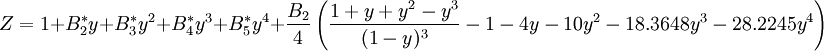$Z = 1+B_2^*y + B_3^*y^2 + B_4^*y^3 + B_5^*y^4 + \frac{B_2}{4} \left( \frac{1+y+y^2-y^3}{(1-y)^3} -1 -4y -10y^2 -18.3648y^3 - 28.2245y^4 \right)$

where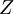$Z$ is the compressibility factor and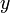$y$ is the volume fraction, given by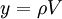$y= \rho V$ where$\rho$ is the number density. The virial coefficients are given by the fits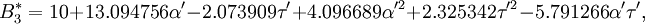$B_3^* = 10 + 13.094756 \alpha' - 2.073909\tau' + 4.096689 \alpha'^2 + 2.325342\tau'^2 - 5.791266\alpha' \tau',$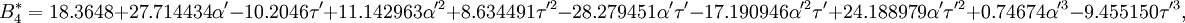$B_4^* = 18.3648 + 27.714434\alpha' - 10.2046\tau' + 11.142963\alpha'^2 + 8.634491\tau'^2 - 28.279451\alpha' \tau' - 17.190946\alpha'^2 \tau' + 24.188979\alpha' \tau'^2 + 0.74674\alpha'^3 - 9.455150\tau'^3,$

and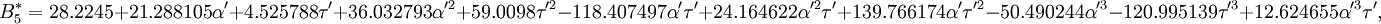$B_5^* = 28.2245 + 21.288105\alpha' + 4.525788\tau' + 36.032793\alpha'^2 + 59.0098\tau'^2 - 118.407497\alpha' \tau' + 24.164622\alpha'^2 \tau' + 139.766174\alpha' \tau'^2 - 50.490244\alpha'^3 - 120.995139\tau'^3 + 12.624655\alpha'^3\tau',$

where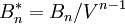$B_n^*= B_n/V^{n-1}$,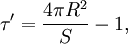$\tau' = \frac{4 \pi R^2}{S} -1,$

and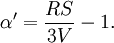$\alpha' = \frac{RS}{3V}-1.$

where$V$ is the volume,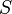$S$, the surface area, and$R$ the mean radius of curvature. These can be calculated using this Mathematica notebook file for calculating the surface area and mean radius of curvature of an ellipsoid. For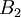$B_2$ see the page "Second virial coefficient".